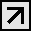UAHReviews: 2
+38 (067) 622-14-60

# Counting scales

In stock
Calculation of the smallest objects in large numbers. Calculating resolution is up to 6 million unitsKERN modelScales for definition of quantity of KFPRange of weighing [Max.], kgPrice of division [d],PlatformscalesControlscalesCFSRangeweighings of [Max.] kgPrice of division [d],Minimum weight of a...
Group: Counting scales
In stock
Industrial calculating scales with the comfortable decimal keyboard for convenient data input, with the calculating resolution of 60 000KERN modelRange of weighing [Max.], kgPrice of division [d],Minimum weight of a subject [Account] of g/pieceCalculating permissionWeight unpacked apprx.&n
Group: Counting scales
In stock
Industrial calculating scales with the comfortable decimal keyboard for convenient data input, with the calculating resolution of 60 000KERN modelRange of weighing [Max.], kgPrice of division [d],Minimum weight of a subject [Account] of g/pieceCalculating permissionWeight unpacked apprx.&n
Group: Counting scales
In stock
Industrial calculating scales with the comfortable decimal keyboard for convenient data input, with the calculating resolution of 60 000KERN modelRange of weighing [Max.], kgPrice of division [d],Minimum weight of a subject [Account] of g/pieceCalculating permissionWeight unpacked apprx.&n
Group: Counting scales
In stock
Industrial calculating scales with the comfortable decimal keyboard for convenient data input, with the calculating resolution of 60 000KERN modelRange of weighing [Max.], kgPrice of division [d],Minimum weight of a subject [Account] of g/pieceCalculating permissionWeight unpacked apprx.&n
Group: Counting scales
In stock
Industrial calculating scales with the comfortable decimal keyboard for convenient data input, with the calculating resolution of 60 000KERN modelRange of weighing [Max.], kgPrice of division [d],Minimum weight of a subject [Account] of g/pieceCalculating permissionWeight unpacked apprx.&n
Group: Counting scales
In stock
Industrial calculating scales with the comfortable decimal keyboard for convenient data input, with the calculating resolution of 60 000KERN modelRange of weighing [Max.], kgPrice of division [d],Minimum weight of a subject [Account] of g/pieceCalculating permissionWeight unpacked apprx.&n
Group: Counting scales
In stock
Industrial calculating scales with the comfortable decimal keyboard for convenient data input, with the calculating resolution of 60 000KERN modelRange of weighing [Max.], kgPrice of division [d],Minimum weight of a subject [Account] of g/pieceCalculating permissionWeight unpacked apprx.&n
Group: Counting scales
In stock
The model of professional calculating scales for beginners, with the certificate of checking [M], calculating resolution is 30 000 unitsKERN modelRange of weighing [Max.], kgPrice of division [d],Parameter of checking [e] ofMin. loading [Min.] ofMinimum weight of a subject [Account]...
Group: Counting scales
In stock
The model of professional calculating scales for beginners, with the certificate of checking [M], calculating resolution is 30 000 unitsKERN modelRange of weighing [Max.], kgPrice of division [d],Parameter of checking [e] ofMin. loading [Min.] ofMinimum weight of a subject [Account]...
Group: Counting scales
In stock
The model of professional calculating scales for beginners, with the certificate of checking [M], calculating resolution is 30 000 unitsKERN modelRange of weighing [Max.], kgPrice of division [d],Parameter of checking [e] ofMin. loading [Min.] ofMinimum weight of a subject [Account]...
Group: Counting scales
In stock
Calculation of the smallest objects in large numbers. Calculating resolution is up to 6 million unitsKERN modelScales for definition of quantity of KFPRange of weighing [Max.], kgPrice of division [d],PlatformscalesControlscalesCFSRangeweighings of [Max.] kgPrice of division [d],Minimum weight of a...
Group: Counting scales
In stock
Calculation of the smallest objects in large numbers. Calculating resolution is up to 6 million unitsKERN modelScales for definition of quantity of KFPRange of weighing [Max.], kgPrice of division [d],PlatformscalesControlscalesCFSRangeweighings of [Max.] kgPrice of division [d],Minimum weight of a...
Group: Counting scales
In stock
Calculation of the smallest objects in large numbers. Calculating resolution is up to 6 million unitsKERN modelScales for definition of quantity of KFPRange of weighing [Max.], kgPrice of division [d],PlatformscalesControlscalesCFSRangeweighings of [Max.] kgPrice of division [d],Minimum weight of a...
Group: Counting scales
In stock
Calculation of the smallest objects in large numbers. Calculating resolution is up to 6 million unitsKERN modelScales for definition of quantity of KFPRange of weighing [Max.], kgPrice of division [d],PlatformscalesControlscalesCFSRangeweighings of [Max.] kgPrice of division [d],Minimum weight of a...
Group: Counting scales## Description

Unbelievable price on Counting scales in Dnipro (Ukraine) company Venta Lab. Wide choice of quality products at affordable prices.